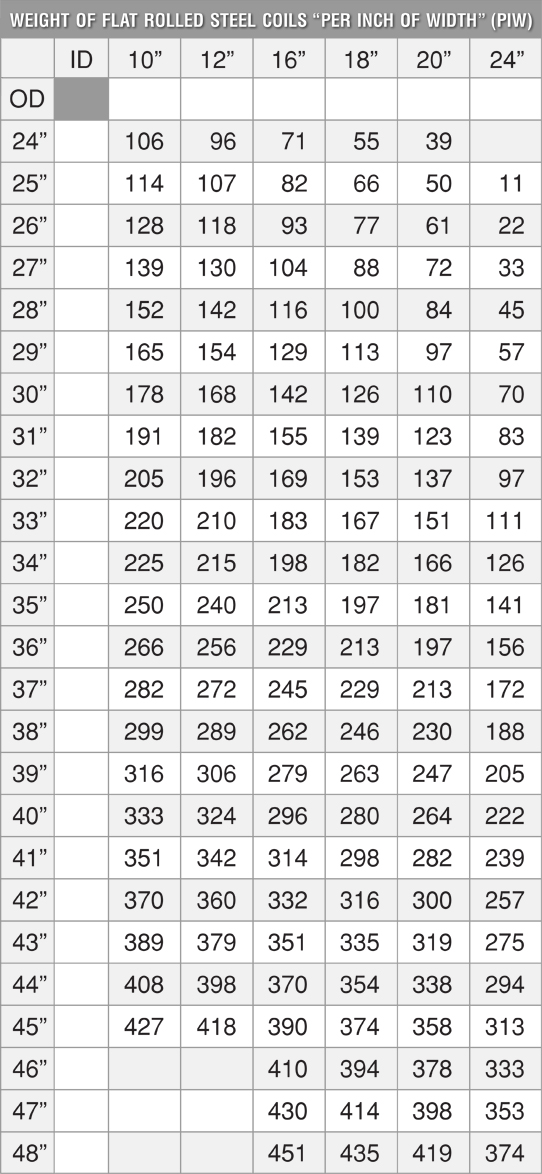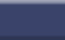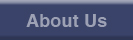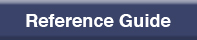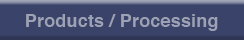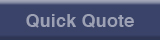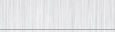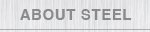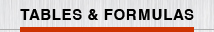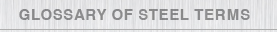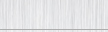| WEIGHT TABLES | FORMULAS |For other sizes: (OD sq - ID sq) x .2223 - weight PIW For footage in coil: weight PIW divided by weight per lineal foot Weight per lineal ft: thickness (decimals) x width x 3.4 Weight Blank: decimal thick x width x .2833 x length (in inches) To convert weights to stainless: 400 series above weight x 1.01 300 series: Above weight x 1.03 Footage per coil: Weight per coil divided by weight per lineal foot.